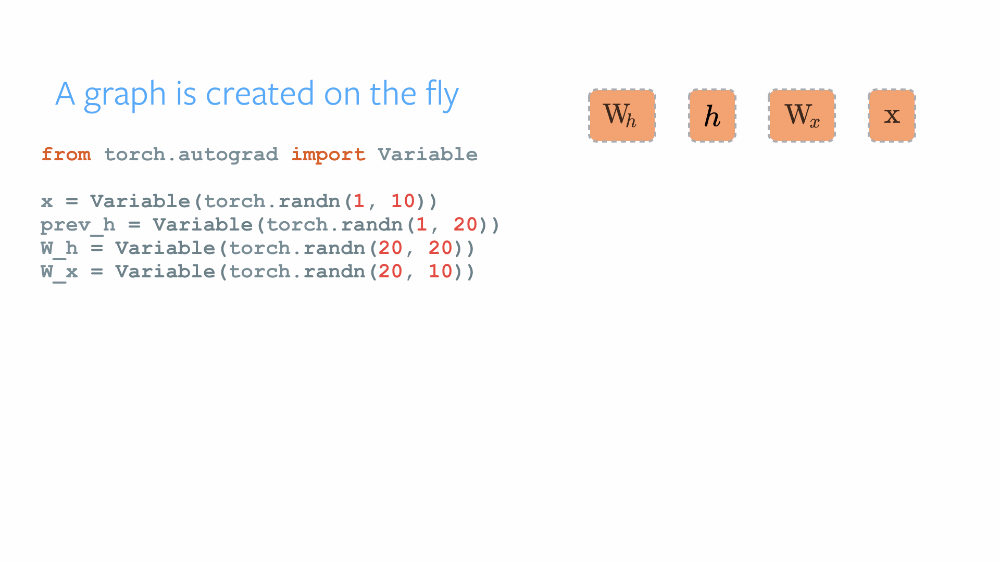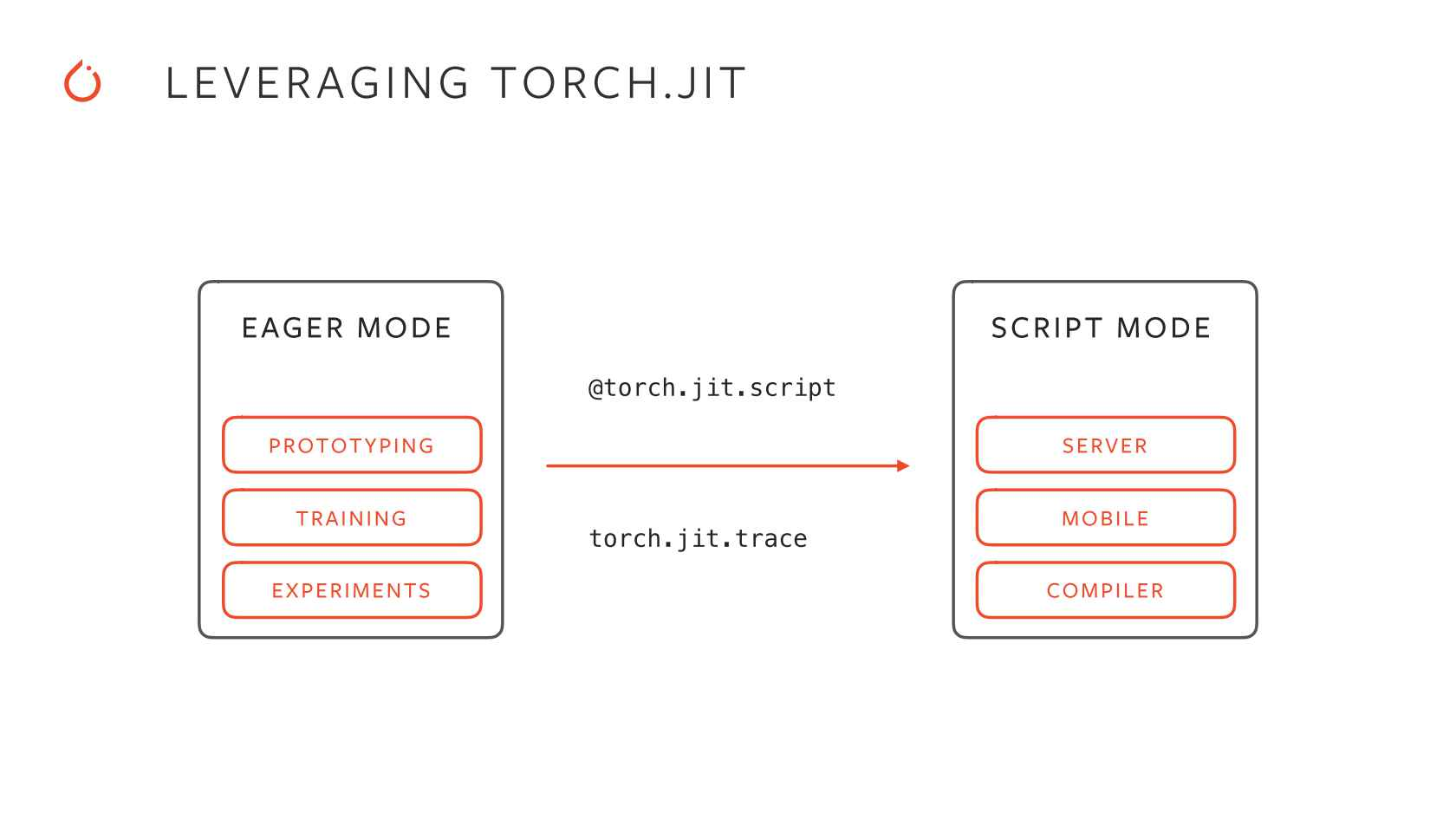# Root-Project: PyTorch Parser for SOFIE

## Translating a .pt file to SOFIE's RModel

Sanjiban Sengupta
·Aug 22, 2021·Post implementing the Keras Parser for SOFIE's RModel, I started developing the PyTorch Parser. PyTorch supports Native export to the `.onnx` format and thus has an internal mechanism to trace the ONNX operators used in a PyTorch model. PyTorch, a rapidly growing Deep Learning framework, is supported in ROOT TMVA via the PyRoot Interface for Python bindings. For saving and importing a saved model, ROOT uses TorchScript.

In this blog of my GSoC'21 with CERN-HSF Series, I discuss the implementation of the PyTorch Parser for translating a `.pt` file into SOFIE's RModel for the subsequent generation of Inference code.

## 1. PyTorch & TorchScript

As the official repository of PyTorch describes it as a Framework for Tensors and Dynamic neural networks in Python with strong GPU acceleration, PyTorch provides a robust Python-based platform with a C++ backend based on Torch, for implementing neural networks and conducting tensor-based high-level computations. The open-source framework was developed by Facebook and later was made public. Having an imperative approach, PyTorch maintains a Dynamic Computational Graph for defining the order of computations and calculating gradients for the neural networks' gradient descent optimization. The computational graph is built dynamically as and when the variables are declared. Thus, after each successive training iteration, this graph is built again. Its dynamic nature makes it more flexible and easy to debug. TensorFlow maintained a Static Computational Graph before the advent of TensorFlow2 Alpha. The main difference in these paradigms can be understood by various analogies. TensorFlow following the " define and run" philosophy works like any compiler where all the variables are defined, connected, initialized in the graph first, and are then reused, but the internal structure cannot be rebuilt again in the go. PyTorch's following the "define and run" concept, thus the DCG works like an interpreter, where the graph is built in every step of the process, and its internals can be modified at runtime.### TorchScript

Unlike Keras & TensorFlow's `model.save(filename)` to save the complete deep learning model with both its configuration and weights, saving a model using PyTorch's `torch.save()` bounds the serialized data to the specific classes and the directory structure, thus the model class is required to be defined before loading the model.

TorchScript provides the functionality to optimize PyTorch models and serialize them from a Python process which can later be loaded in environments not necessarily dependent on Python, like a C++ standalone program. The addition of TorchScript to PyTorch implements a unified framework for transitioning models from research to production. Serializing a PyTorch module to TorchScript lets us save the entire model with the configuration and weights which can later be used for inference. TorchScript provides two methods of execution namely `Trace` and `Script`. Tracing a model requires an input tensor which is passed and the computations operating on the tensor in its way until the output are recorded, and thus can be used later for input tensor of similar shapes. Scripting a model is more generalized where the entire model is all of its configurations is serialized and can be used later with tensors of any applicable shapes.Root TMVA's PyTorch interface allows training the PyTorch models in the TMVA environment and serializing them using TorchScript which can later be used for inference.

## 2. Parsing PyTorch `.pt` files

Because of the flexibility that PyTorch provides in building a neural network model with dynamic addition of model computations in the graph, it's difficult to extract the information of the layers included, so as to parse the configuration and build the RModel object. PyTorch models can be built in `nn.Sequential`, `nn.Module` and `nn.ModuleList` containers and also supports encapsulating layers into units for subclassing. As ROOT TMVA's PyTorch interface saves and loads a scripted module of the model using TorchScript, which just contains the model routines and weights for inference, thus extracting the ground-level features that make up a PyTorch model is difficult unlike a Keras Sequential API and Functional API model, where the graph can be traversed iteratively.

To solve this and find a way for the extraction of the included layers and their attributes, we can take the help of the Native ONNX support that PyTorch provides.

PyTorch has internal implementations which convert the model into its ONNX equivalent graph. For this, a dummy input tensor is required which is passed through the model and the applied operators in its course are recorded for developing the ONNX graph. While using a saved script module for this, we also require expected example outputs for shape inference. For working on tensors with dynamic axes, we again use a PyTorch internal functionality which sets the shape inference for them.

``````#Converting the model to ONNX Graph representation
import torch
from torch.onnx.utils import _model_to_graph
from torch.onnx.symbolic_helper import _set_onnx_shape_inference

model.eval()
model.float()

#Building the dummy input tensor
s1=[120,1]
dummy=torch.rand(*s1)
input=[dummy]
output=model(*input)

#Setting inference for dynamic axes
_set_onnx_shape_inference(True)

#Generating the equivalent ONNX graph
graph=_model_to_graph(model,input,example_outputs=output)
``````

The output of the graph looks something like this

``````>>> graph
(graph(%x.1 : Float(2, 6, strides=[6, 1], requires_grad=0, device=cpu),
%fc1.weight : Float(12, 6, strides=[6, 1], requires_grad=0, device=cpu),
%fc1.bias : Float(12, strides=, requires_grad=0, device=cpu)):
%3 : Float(2, 12, strides=[12, 1], device=cpu) = onnx::Gemm[alpha=1., beta=1., transB=1](%x.1, %fc1.weight, %fc1.bias)
%4 : Float(2, 12, strides=[12, 1], device=cpu) = onnx::Relu(%3)
%5 : Float(12, 2, strides=[1, 12], requires_grad=1, device=cpu) = onnx::Transpose[perm=[1, 0]](%4)
return (%5)
, {'fc1.bias': tensor([ 0.1858, -0.4581, -0.3743, -0.0609, -0.1052,  0.0538,  0.0467, -0.1544,
-0.3622, -0.3611, -0.2269,  0.1716]), 'fc1.weight': tensor([[ 1.9536e-01,  3.3694e-02, -9.5294e-02, -3.2092e-01, -2.6642e-01,
3.2872e-01],
[ 2.5622e-01, -2.5419e-01,  3.2304e-01, -5.7926e-02,  1.8545e-01,
-3.3965e-01],
[ 2.3855e-01, -3.0029e-01, -3.2645e-01, -3.6467e-01, -1.1971e-01,
-1.5546e-01],
[-3.9777e-01, -2.4680e-01,  4.6634e-02,  7.5872e-05,  5.0814e-02,
-3.0577e-01],
[ 9.8371e-03, -3.5033e-01, -1.8954e-01,  2.4746e-01, -1.9828e-01,
-3.3955e-01],
[-2.3662e-02, -3.1937e-02, -8.0010e-02,  1.6615e-01,  2.1353e-01,
-3.5624e-01],
[-1.0537e-01, -1.0636e-01,  3.1500e-01, -2.0943e-01,  6.0947e-01,
2.3454e-01],
[-1.7886e-01, -1.6449e-01, -7.1962e-02, -1.4553e-01,  2.7605e-01,
2.8320e-02],
[-4.0435e-01,  3.2550e-01, -2.1856e-01, -3.2801e-02, -3.6782e-01,
-2.4415e-01],
[ 1.9953e-01,  2.1276e-01, -6.8311e-02,  7.6783e-02,  1.1836e-01,
1.2552e-01],
[ 1.5363e-01, -2.6658e-01, -4.5881e-01, -2.5509e-01,  1.9992e-01,
6.6146e-02],
[-3.3991e-01, -1.3903e-01, -1.1859e-01, -8.4289e-02,  1.4896e-02,
1.6103e-01]])}, None)
``````

Here graph is a tuple, where `graph` holds the model configuration and `graph` holds the model weights. As the graph is of ONNX graph format, so we can directly iterate through it and extract the node data.

``````modelData = []

for i in graph.nodes():
globals().update(locals())

#For type of node
nodeData = []
nodeData.append(i.kind())

#For attrbutes of node
nodeAttributeNames = [x for x in i.attributeNames()]
nodeAttributes     = {j:i[j] for j in nodeAttributeNames}
nodeData.append(nodeAttributes)

#For input tensor info of node
nodeInputs     = [x for x in i.inputs()]
nodeInputNames = [x.debugName() for x in nodeInputs]
nodeData.append(nodeInputNames)

#For output tensor info of node
nodeOutputs     = [x for x in i.outputs()]
nodeOutputNames = [x.debugName() for x in nodeOutputs]
nodeData.append(nodeOutputNames)

modelData.append(nodeData)
``````

This extracted data is then used in a similar way as the Keras parser to add the operators, input & output tensor infos, and initialized tensors into the RModel object for successful translation. Root being a framework primarily developed in C++, thus the C-Python API was used for all these extractions and manipulations of Python variables. The official C-Python API can be used for understanding the usage, with a simpler guide from IBM's DeveloperWorks which can be found here.

The development of the PyTorch Parser for SOFIE can be tracked with PR#8684.

## 3. Tests

Tests were developed for validating the implemented parser, which was similar to the ones for the Keras parser. Python scripts are developed which are run from the C-Python API to generate and save a PyTorch model, and then this model is parsed into a RModel object, and subsequently, the inference code is generated. The correctness is then found on Google's GTest framework by comparing the outputs of the PyTorch model and from the generated inference code when called on the same input tensors.

This marked the completion of implementing the PyTorch parser for SOFIE and with this, my major tasks for my Google Summer of Code Project were completed. With time remaining in the official coding period, I also started developing the Root-Storage for BDT models, for their serialization and inference code generation. Post-GSoC, I will be describing the development of the Root-Storage of BDT when a substantial part of the functionality would have been implemented, and my following blog will be on the final report for GSoC concluding and summarizing this amazing journey of 10 weeks.